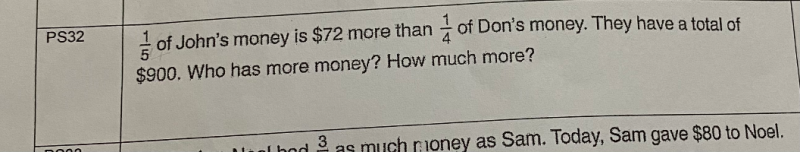# QuestionLet u be  Don’s 1/4 money and hence John’s 1/5 money is (u+72)

Partial Amount              Total Amount for Each                Total for Both of them

Don    [u]                                                       4u

900

John   [u]                                         5(u+72)

4u + 5(u+72) = 900

4u + 5u+ 360 = 900

9u = 540

u = 60

Don’s money = 4 x 60 = 240

John’s money = 5(60+72) = 660

Difference = 660-240 = 420

Hence John has \$420 more than Don.

0 Replies 1 Like ✔Accepted Answer